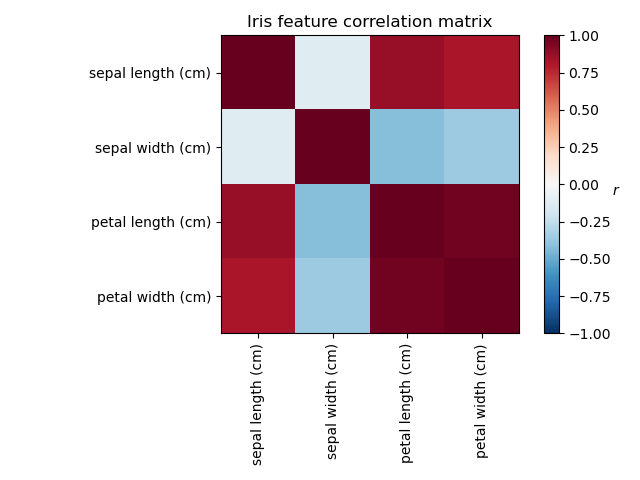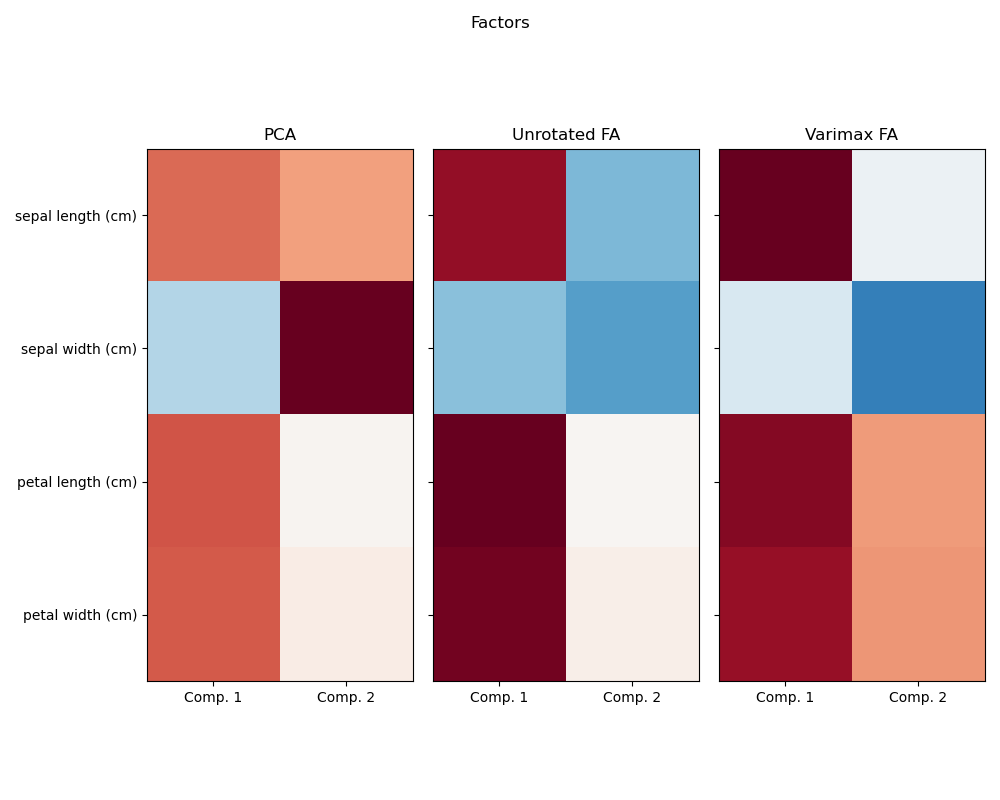# Factor Analysis (with rotation) to visualize patterns¶

Investigating the Iris dataset, we see that sepal length, petal length and petal width are highly correlated. Sepal width is less redundant. Matrix decomposition techniques can uncover these latent patterns. Applying rotations to the resulting components does not inherently improve the predictve value of the derived latent space, but can help visualise their structure; here, for example, the varimax rotation, which is found by maximizing the squared variances of the weights, finds a structure where the second component only loads positively on sepal width.

# Authors: Jona Sassenhagen

import matplotlib.pyplot as plt
import numpy as np

from sklearn.decomposition import FactorAnalysis, PCA
from sklearn.preprocessing import StandardScaler

print(__doc__)


data = load_iris()
X = StandardScaler().fit_transform(data["data"])
feature_names = data["feature_names"]


Plot covariance of Iris features

ax = plt.axes()

im = ax.imshow(np.corrcoef(X.T), cmap="RdBu_r", vmin=-1, vmax=1)

ax.set_xticks([0, 1, 2, 3])
ax.set_xticklabels(list(feature_names), rotation=90)
ax.set_yticks([0, 1, 2, 3])
ax.set_yticklabels(list(feature_names))

plt.colorbar(im).ax.set_ylabel("$r$", rotation=0)
ax.set_title("Iris feature correlation matrix")
plt.tight_layout()Run factor analysis with Varimax rotation

n_comps = 2

methods = [('PCA', PCA()),
('Unrotated FA', FactorAnalysis()),
('Varimax FA', FactorAnalysis(rotation='varimax'))]
fig, axes = plt.subplots(ncols=len(methods), figsize=(10, 8))

for ax, (method, fa) in zip(axes, methods):
fa.set_params(n_components=n_comps)
fa.fit(X)

components = fa.components_.T
print("\n\n %s :\n" % method)
print(components)

vmax = np.abs(components).max()
ax.imshow(components, cmap="RdBu_r", vmax=vmax, vmin=-vmax)
ax.set_yticks(np.arange(len(feature_names)))
if ax.is_first_col():
ax.set_yticklabels(feature_names)
else:
ax.set_yticklabels([])
ax.set_title(str(method))
ax.set_xticks([0, 1])
ax.set_xticklabels(["Comp. 1", "Comp. 2"])
fig.suptitle("Factors")
plt.tight_layout()
plt.show()Out:

 PCA :

[[ 0.52106591  0.37741762]
[-0.26934744  0.92329566]
[ 0.5804131   0.02449161]
[ 0.56485654  0.06694199]]

Unrotated FA :

[[ 0.88096009 -0.4472869 ]
[-0.41691605 -0.55390036]
[ 0.99918858  0.01915283]
[ 0.96228895  0.05840206]]

Varimax FA :

[[ 0.98633022 -0.05752333]
[-0.16052385 -0.67443065]
[ 0.90809432  0.41726413]
[ 0.85857475  0.43847489]]


Total running time of the script: ( 0 minutes 0.398 seconds)

Gallery generated by Sphinx-Gallery